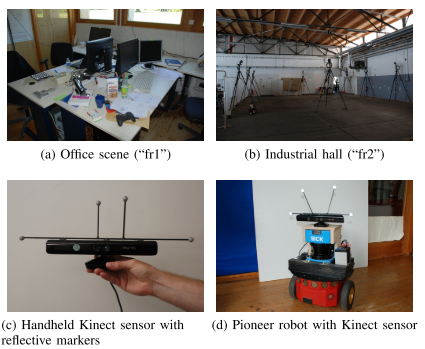# 2012-A benchmark for the evaluation of RGB-D SLAM systems 视觉SLAM：算法精度评价指标（ATE、RPE）**数据集格式** All data was recorded at full resolution (640×480) and full frame rate (30 Hz) of the Microsoft Xbox Kinect sensor. - “rgb/”: a folder containing all color images (PNG for- mat, 3 channels, 8 bit per channel), - “depth/”: same for the depth images (PNG format, 1 channel, 16 bit per channel, distance in meters scaled by factor 5000), - “rgb.txt”: a text file with a consecutive list of all color images (format: timestamp filename), - “depth.txt”: same for the depth images (format: times- tamp filename), - “imu.txt”: a text file containing the timestamped ac- celerometer data (format: timestamp fx fy fz), - “groundtruth.txt”: a text file containing the ground truth trajectory stored as a timestamped translation vector and unit quaternion (format: timestamp tx ty tz qx qy qz qw). **EVALUATION METRICS** The estimated trajectory: $P_1,...,P_n \in SE(3)$ The ground truth trajectory: $Q_1,...,Q_n \in SE(3)$ A fixed time interval $\Delta$ **RPE: relative pose error 相对位姿误差** We define the relative pose error at time step i as $E_i:=(Q^{-1}Q_{i+\Delta})^{-1}(P_i^{-1}P_{i+\Delta})$ From a sequence of n camera poses, we obtain in this way m = n − ∆ individual relative pose errors along the sequence. From these errors, we propose to compute the root mean squared error (RMSE) over all time indicies of the translational component as: $RMSE(E_{1:n},\Delta):=(\frac1m \sum_{i=1}^m || trans(E_i)||^2)^{\frac12}$ For the evaluation of SLAM systems, it therefore makes sense to average over all possible time intervals $\Delta$: $RMSE(E_{1:n}):=\frac1n\sum_{\Delta=1}^n \text{RMSE}(E_{1:n},\Delta)$ **ATE: absolute trajectory error 绝对轨迹误差** The global consistency can be evaluated by comparing the absolute distances between the estimated and the ground truth trajectory. S corresponding to the least-squares solution that maps the estimated trajectory P1:n onto the ground truth trajectory Q1:n. Given this transformation, the absolute trajectory error at time step i can be computed as: $F_i:=Q_i^{-1}SP_i$ where $S\in Sim(3)$ we propose to evaluate the root mean squared error over all time indices of the translational components, i.e., $RMSE(F_{1:n},\Delta):=(\frac1n \sum_{i=1}^n || trans(F_i) || ^2)^{\frac12}$ The RPE can be used to evaluate the global error of a trajectory by averaging over all possible time intervals. Note that the RPE considers both translational and rotational errors, while the ATE only considers the translational errors. RPE 既考虑旋转又考虑位移误差，而ATE只考虑位移误差 # EVO TOOLS This package provides executables and a small library for handling, evaluating and comparing the trajectory output of odometry and SLAM algorithms. Supported trajectory formats: - 'TUM' trajectory files - 'KITTI' pose files - 'EuRoC MAV' (.csv groundtruth and TUM trajectory file) - ROS bagfile with geometry_msgs/PoseStamped, geometry_msgs/TransformStamped, geometry_msgs/PoseWithCovarianceStamped or nav_msgs/Odometry topics or TF messages Github: https://github.com/MichaelGrupp/evo ![Error mapped onto trajectory](https://raw.githubusercontent.com/MichaelGrupp/evo/master/doc/assets/ape_demo_ORB_map.png) Installation: sh pip install evo --upgrade --no-binary evo  # References - Sturm, J., Engelhard, N., Endres, F., Burgard, W., & Cremers, D. (2012). A benchmark for the evaluation of RGB-D SLAM systems. IEEE International Conference on Intelligent Robots and Systems, 573–580. https://doi.org/10.1109/IROS.2012.6385773 - [RGB-D SLAM Dataset and Benchmar](https://vision.in.tum.de/data/datasets/rgbd-dataset) - [MichaelGrupp/evo](https://github.com/MichaelGrupp/evo)